# Class 12 Maths NCERT Solutions for Chapter 4 Determinants Exercise 4.2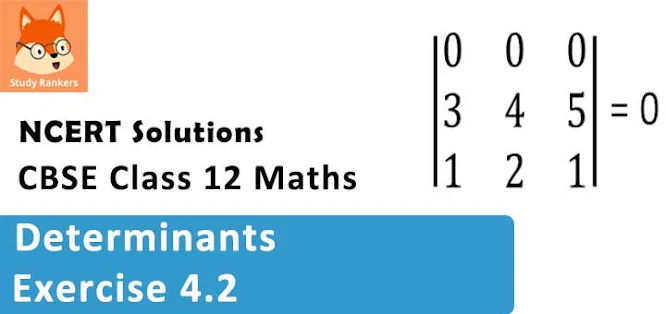### Determinants Exercise 4.2 Solutions

1. Using the property of determinants and without expanding,  prove that :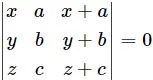Solution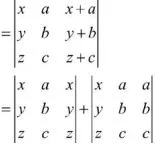Here, two columns of each determinant are identical.

2. Using the property of determinants and without expanding, prove that :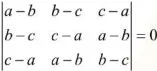Solution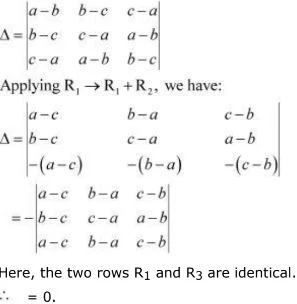3. Using the property of determinants and without expanding, prove that :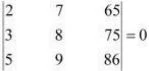Solution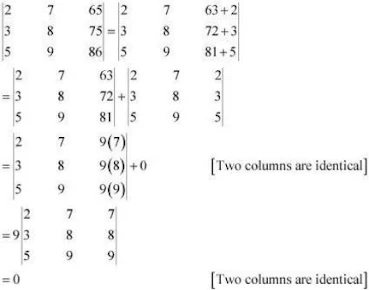4. Using the property of determinants and without expanding, prove that :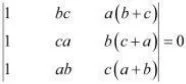Solution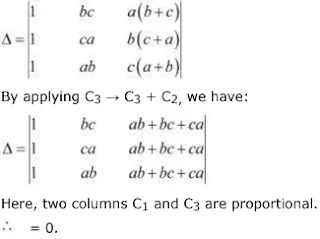5. Using the property of determinants and without expanding, prove that :Solution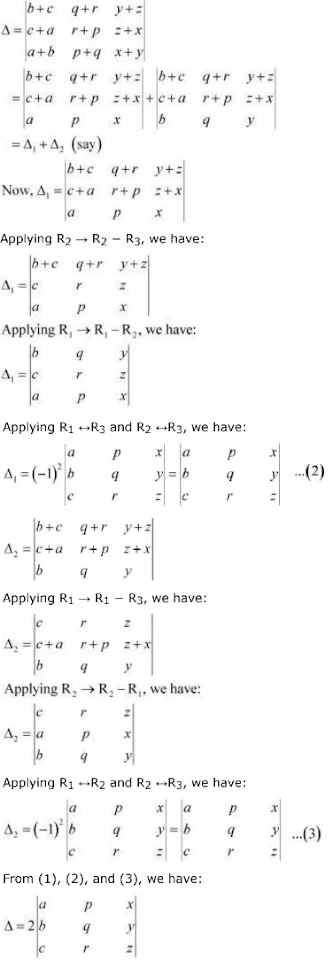Hence, the given result is proved.

6. By using properties of determinants, show that: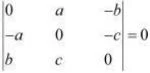Solution
We have,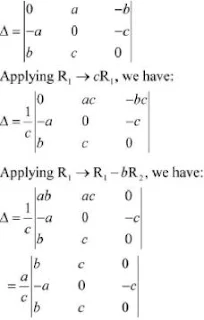Here, the two rows R1 and R3 are identical.
∴ Δ = 0.

7. By using properties of determinants, show that :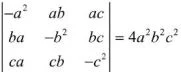Solution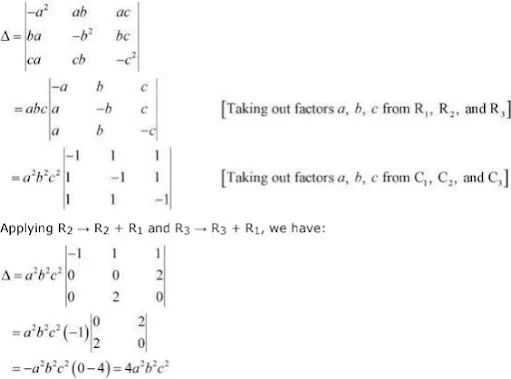8. By using properties of determinants, show that :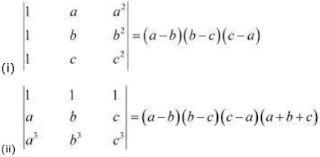Solution
(i) Let Δ =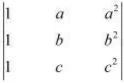Applying R1 → R1 → R3 and R2 → R2 →R3 , we have :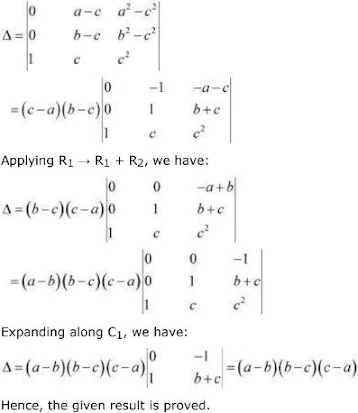(ii) Let  Δ =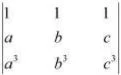Applying C1 → C1 → C3 and C2 → C2 →C3 , we have :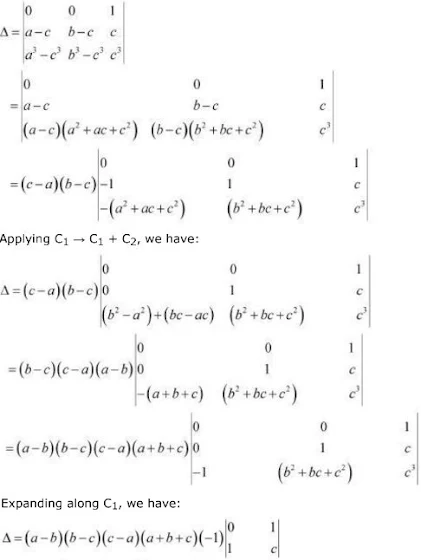= (a - b)(b - c)(c - a)(a + b + c)
Hence, the given result is proved.

9. By using properties of determinants, show that :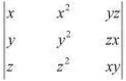= (x - y)(y - z)(z - x)(xy + yz + zx)

Solution

Let Δ =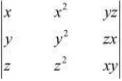Applying R2 → R2 → R1 and R3 → R3 → R1 , we have :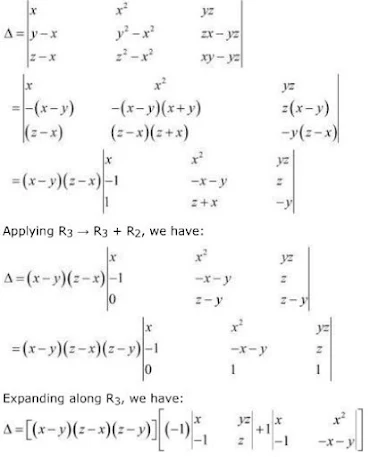= (x - y)(z - x)(z- y)[(-xz - yz) + (-x2 – xy + x2 )]
= -(x - y)(z -x)(z - y)(xy + yz + zx)
= (x - y)(y - z)(z - x)(xy + yz + zx)
Hence, the given result is proved.

10. By using properties of determinants, show that :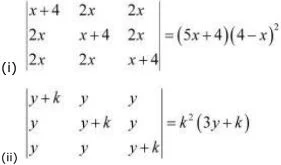Solution

(i)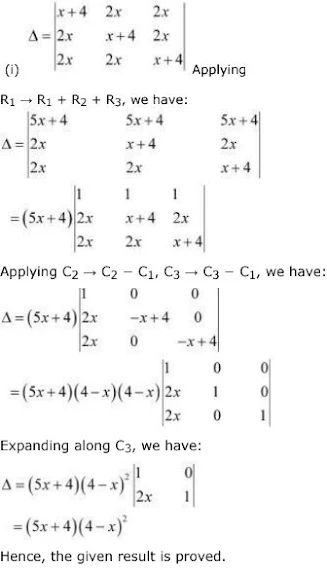(ii)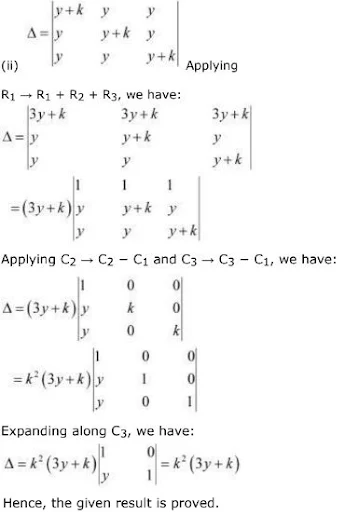11. By using properties of determinants, show that :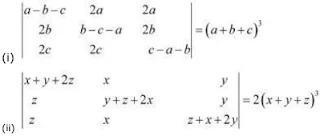Solution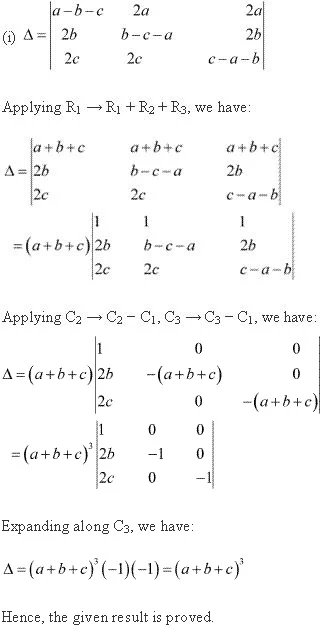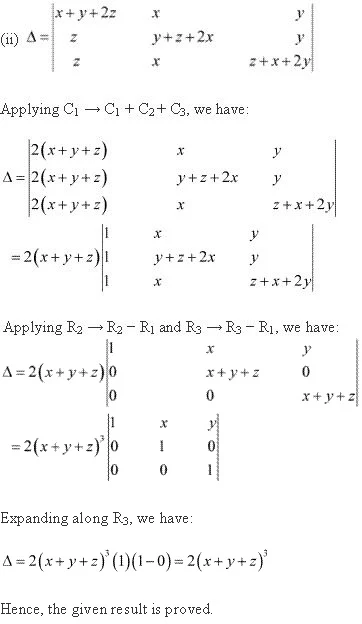12. By using properties of determinants show that :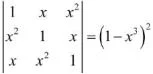Solution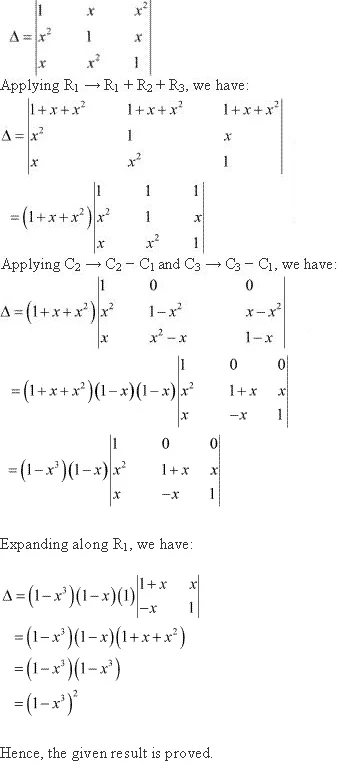13. By using properties of determinants show that :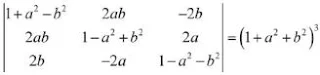Solution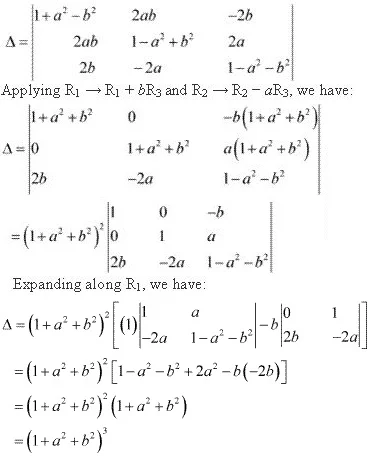14. By using properties of determinants show that :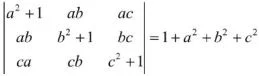Solution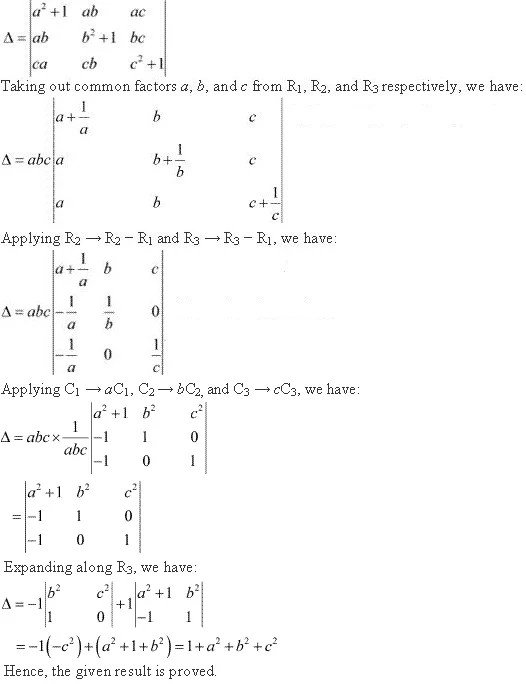15. Let A be a square matrix of order 3 × 3, then | kA| is equal to
(A) k|A|
(B) k2 | A |
(C) k3 | A |
(D) 3k | A |
Solution
Let A =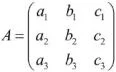Then,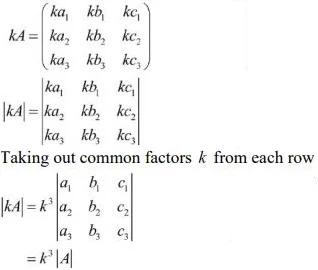The correct option is C.

16. Which of the following is correct?
(A) Determinant is a square matrix.
(B) Determinant is a number associated to a matrix.
(C) Determinant is a number associated to a square matrix.
(D) None of the above.
Solution
We know that to every square matrix, A = [aij] of order n , we can associate a number called the determinant of square matrix A, where aij = (i, j)th element of A. Thus, the determinant is a number associated to a square matrix. Hence, the correct option is C.